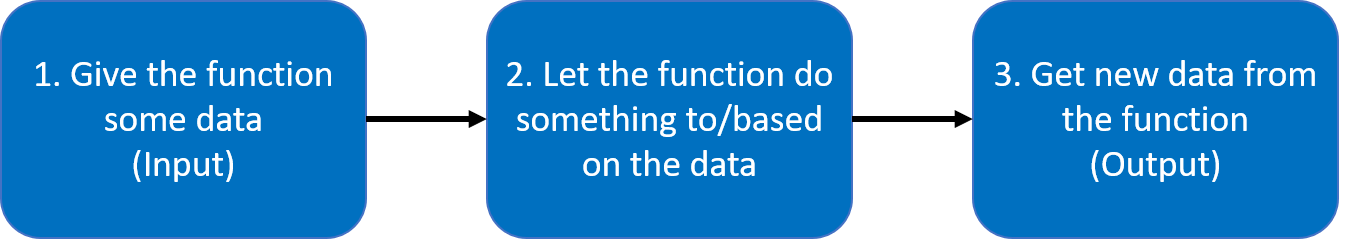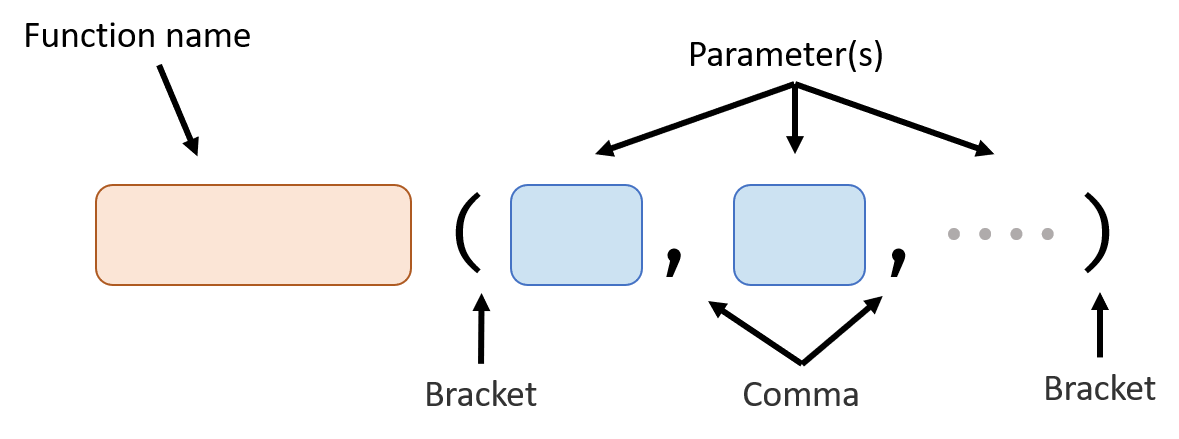# Basic Function

A function performs a per-defined task when you use it (also known as “when you call it”).

Now, we can only use functions that are pre-defined by python. In the Advanced Function handout, we will learn how to define a function by ourselves.

Here’s the full process of using a function:## Basic Structure### Parameter(s)

This is the place where you give data to the function.

You can don’t give a function any parameter, one parameter or multiple parameters. Parameters are separated by commas.

For example: (The following code is just for demonstrate how to give data to a function, it can’t actually run because those functions are not defined yet.)

### Return Value

This is how you get new data from the function.

A function can return no value or one value. You can assign the value returned from a function to a variable.

For example: (The following code is just for demonstrate how to get value returned from a function, it can’t actually run because those functions are not defined yet.)

## How to Describe a Function

Knowing how to describe a function is very important when you are trying to figure out how to use a function.

1. Signature

Signature of the function is the function name and the parameter it takes. For example, the signature of the print function is print (data)

2. Parameters

Explain what does each parameter means. For example, data parameter means the data print function will show in the shell.

3. Return value

Describe what and how many value the function will return. For print function, it returns no value.

## print Function

• Usage: output a value

• Signature: print (data)

• Parameters:

• Return value: None

## str Function

• Usage: convert a number type value to string type value

• Signature: str (number)

• Parameters:

• Return value: same number, but is in string type.

Next: Control Flow